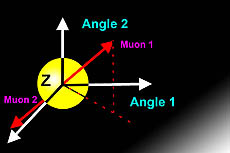## High-precision tests of the Standard Model begin at LHC

May 14, 2015Two Rochester physicists, Professor Arie Bodek and Research Associate Jiyeon Han, helped lead a high-statistics study of the decay of the Z boson at the Compact Muon Solenoid (CMS) detector at CERN. This work, submitted to Physics Letters B in April and highlighted this week at the Fermilab Today newsletter, provides measurements of unprecedented precision of the decay of the Z. The data disagree with theoretical expectations, likely because of insufficiently precise theoretical calculations. As a result, the study has generated great interest among theoretical physicists and will motivate more precision measurements at the Large Hadron Collider (LHC).

The Z boson is one of the best studied of the subatomic particles. Z bosons decay in many ways, but one of the cleanest and easiest to study is when it decays into two muons, which are heavy cousins of electrons. You can do an analysis in any reference frame, from one in which the particle is moving to one that is stationary. If you do your analysis properly, you will draw the same conclusions in any frame. So Bodek, Han, and other CMS scientists picked the easiest frame in which the Z boson was stationary. In such a frame, only a few properties define the particle. The Z boson is electrically neutral and massive. It also decays only via the weak nuclear force. Given the measured mass of the Z boson and decay muons, many of the details of how the muons are emitted are determined completely via energy and momentum conservation. The muons are emitted back to back and with a specific energy.

The one thing that is not predetermined is the angle at which the muon pair is emitted. That angle is related to the spin of the Z boson. The Z boson has spin (which has a complex meaning in the quantum world). Also, the angle is influenced by the nature of the weak force, which prefers certain spins over others.

To simplify the analysis, the experimenters expressed the various possible angular distributions using trigonometric functions. It turns out that you can describe the expected distribution using five functions, and the analysis reduces to five simple numbers that say just how much of each function is needed to describe the data. The analyzers then determined these five numbers and plotted how they changed as a function of the momentum and angle of the Z boson in the reference frame of the LHC collision.

Analyses of this kind are a crucial precursor to doing precision measurements at the LHC, and theoretical physicists are studying this measurement with great interest.
(Credit: D. Lincoln, FNAL)

References:
- CMS Collaboration, arXiv:1504.03512 [hep-ex], subm. to Phys. Lett. B.
- "Weak Angles", Don Lincoln, Fermilab: http://www.fnal.gov/pub/today/archive/archive_2015/today15-05-08.html